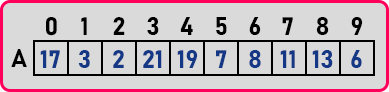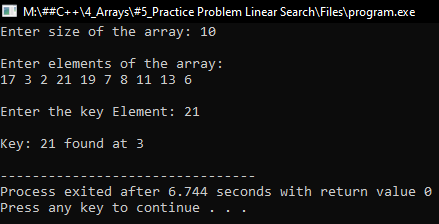# Linear Search in C++

## Linear Search in C++ with Examples:

In this article, I am going to discuss the Linear Search in C++ Language with examples. Please read our previous articles, where we discussed the program for Finding the Max element in an Array using C++ Language with examples.

## Linear Search in C++

1. Linear Search
2. Binary Search

We will study Binary Search in the next article. So, let us understand what is linear search?

##### What is Linear Search?Searching is the process of finding the location of an element. We have an array of size 10. And in this, we want to search for an element. So searching element is usually called a key, we say we’re searching for the key ‘21’.

We have to find out where it is. By looking at the above array, ‘21’ is present at index 5. For example, you have some books on a shelf. They are not sorted. And you are searching for a book. So, you have to look at the books one by one until you find the book that you are searching for. So same process we will adopt here.

##### Process of Linear Search:In the given array, we will start from the 0th index by checking the elements one by one. We want to find ‘21’. So let us start searching. A is ‘17’, move to the next element. A is ‘3’, again moving to the next element.

A is ‘21’. This is the key element that we were searching for. The index for the key ‘21’ is 3. So, we have to scan the array until we reach the index of the key element. Once we got the elements, the search is successful and we stop. Now we’ll take one more key element which is ’14’.

A is ‘17’, it is not ‘14’, move to the next element.

A is ‘3’, again moving to the next element.

A is ‘6’. So, we have reached the end of the array and we didn’t get the element ‘14’. So, the search is unsuccessful. So, the search may be successful or unsuccessful depending on the key that we are searching for. It’s a simple procedure. So let us write a C++ program for that one.

##### Program for Linear Search in C++:
```#include <iostream>
#include <conio.h>
using namespace std;
int main()
{
int n, key;
cout <<"Enter size of the array: ";
cin >> n;
cout << endl;
int A[n];
cout <<"Enter elements of the array:\n";
for (int i = 0; i < n; i++)
{
cin >> A[i];
}

cout <<"\nEnter the key Element: ";
cin >> key;
cout << endl;
for (int i = 0; i < n; i++)
{
if (key == A[i])
{
cout << "Key: " << key << " found at " << i << endl;
return 0;
}
}